# Working with XlsxWriter module – Python

• Last Updated : 22 Nov, 2021

XlsxWriter is a Python module that provides various methods to work with Excel using Python. It can be used to read, write, applying formulas. Also, it supports features such as formatting, images, charts, page setup, auto filters, conditional formatting, and many others.

This tutorial aims at providing knowledge about the XlsxWriter module from basics to advance with the help well explained examples and concepts.

## Installation

Before diving deep into the module let’s start by installing it. To install it type the below command in the terminal.

`pip install XlsxWriter`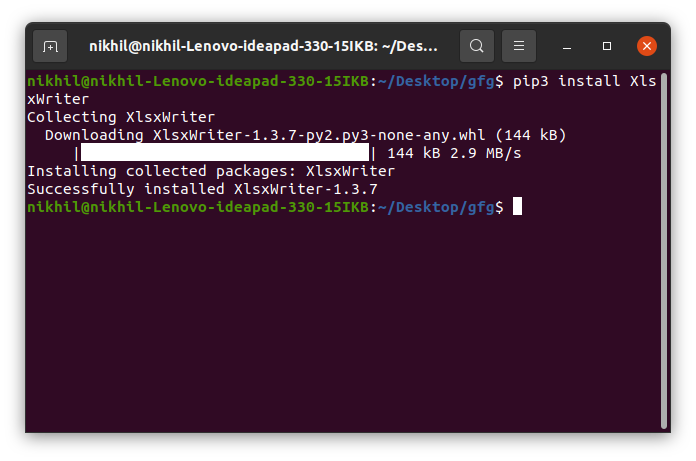You should see the message as successfully installed. Now after the installation let’s dive deep into the module.

## Creating and Writing to Excel

After the installation let’s start by writing a simple code and then we will understand the code.

Example:

## Python3

 `# import xlsxwriter module ``import` `xlsxwriter `` ` `# Workbook() takes one, non-optional, argument ``# which is the filename that we want to create. ``workbook ``=` `xlsxwriter.Workbook(``'sample.xlsx'``) `` ` `# The workbook object is then used to add new ``# worksheet via the add_worksheet() method. ``worksheet ``=` `workbook.add_worksheet() `` ` `# Use the worksheet object to write ``# data via the write() method. ``worksheet.write(``'A1'``, ``'Hello..'``) ``worksheet.write(``'B1'``, ``'Geeks'``) ``worksheet.write(``'C1'``, ``'For'``) ``worksheet.write(``'D1'``, ``'Geeks'``) `` ` `# Finally, close the Excel file ``# via the close() method. ``workbook.close() `

Output: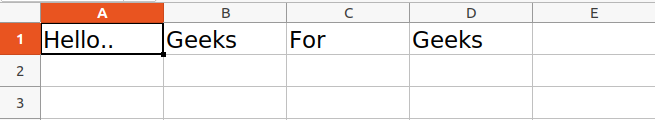In the above example, we have called the function Workbook() which is used for creating an empty workbook. The Excel file will be created with the name of sample.xlsx. Then the add_worksheet() method is used to add a spreadsheet to the workbook and this spreadsheet is saved under the object name worksheet. Then the write() method is used to write the data to the spreadsheet. The first parameter is used to pass the cell name. The cell name can also be passed by the index name such as A1 is indexed as (0, 0), B1 is (0, 1), A2 is (1, 0), B2 is (1, 1).

Note: Rows and Columns are Zero indexed in XlsxWriter.

Now let’s see how to add data to a particular row or column. See the below example.

Example:

## Python3

 `# import xlsxwriter module ``import` `xlsxwriter `` ` `workbook ``=` `xlsxwriter.Workbook(``'sample.xlsx'``) ``worksheet ``=` `workbook.add_worksheet() `` ` `# Start from the first cell. ``# Rows and columns are zero indexed. ``row ``=` `0``column ``=` `0`` ` `content ``=` `[``"Welcome"``, ``"to"``, ``"Geeks"``, ``"for"``, ``"Geeks"``] `` ` `# iterating through content list ``for` `item ``in` `content : `` ` `    ``# write operation perform ``    ``worksheet.write(row, column, item) `` ` `    ``# incrementing the value of row by one ``    ``# with each iterations. ``    ``row ``+``=` `1`` ` `workbook.close()`

Output: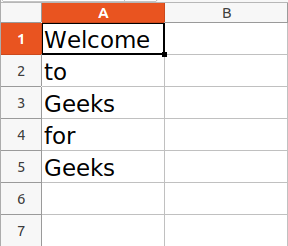You might have seen that we are playing with the index number to write in a particular row. Similarly, we can use a similar way to write to a particular column.

The XlsxWriter module also provides the write_row() and write_column() methods to write in a particular row or column.

Example:

## Python3

 `# import xlsxwriter module ``import` `xlsxwriter `` ` `workbook ``=` `xlsxwriter.Workbook(``'sample.xlsx'``) ``worksheet ``=` `workbook.add_worksheet() `` ` `content ``=` `[``"Welcome"``, ``"to"``, ``"Geeks"``, ``"for"``, ``"Geeks"``] `` ` `# Writing to row and column respectively``worksheet.write_row(``0``, ``1``, content)``worksheet.write_column(``1``, ``0``, content)`` ` `workbook.close()`

Output: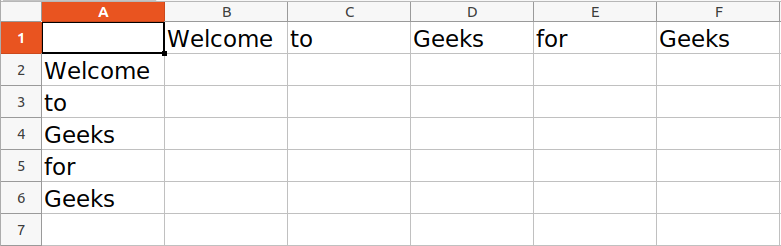Refer to the below article to get detailed information about writing to Excel using the XlsxWriter module.

## Working with Formulas

XlsxWriter module provides the write_formula() and write_array_formula() methods to directly write the formulas in Excel.

• write_formula() method is used to directly write the formula to a worksheet cell
• write_array_formula() method is used to write an array formula to a worksheet cell. Array formula in Excel is that formula that performs on a set of values.

Syntax:

write_formula(row, col, formula[, cell_format[, value]])

write_array_formula(first_row, first_col, last_row, last_col, formula[, cell_format[, value]])

Example 1: Using the write_formula() method

## Python3

 `# import xlsxwriter module ``import` `xlsxwriter `` ` `workbook ``=` `xlsxwriter.Workbook(``'sample.xlsx'``) ``worksheet ``=` `workbook.add_worksheet() `` ` `content ``=` `[``1``, ``2``] `` ` `# Writing to row and column respectively``worksheet.write_row(``0``, ``1``, content)``worksheet.write_column(``1``, ``0``, content)`` ` `# Using the array formula to find the``# sum and the product of the given cells``worksheet.write_formula(``'A4'``, ``'{=SUM(A2, A3)}'``)``worksheet.write_formula(``'D1'``, ``'{=PRODUCT(B1, C1)}'``)`` ` `workbook.close()`

Output: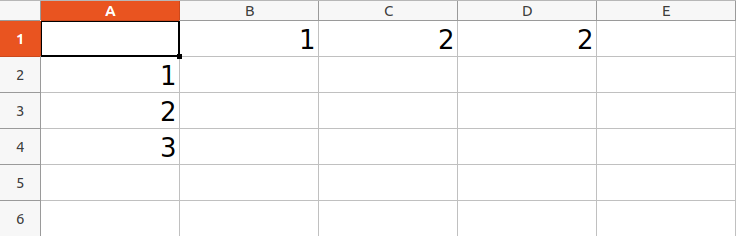Example 2: Using the write_array_formula() method

## Python3

 `# import xlsxwriter module ``import` `xlsxwriter `` ` `workbook ``=` `xlsxwriter.Workbook(``'sample.xlsx'``) ``worksheet ``=` `workbook.add_worksheet() `` ` `content ``=` `[``1``, ``2``, ``3``, ``4``, ``5``] `` ` `# Writing to row and column respectively``worksheet.write_row(``0``, ``1``, content)``worksheet.write_column(``1``, ``0``, content)`` ` `# Using the array formula to find the``# sum and the product of the given cells``worksheet.write_array_formula(``'A7'``, ``'{=SUM(A1:A6)}'``)``worksheet.write_array_formula(``'G1'``, ``'{=PRODUCT(B1:F1)}'``)`` ` `workbook.close()`

Output: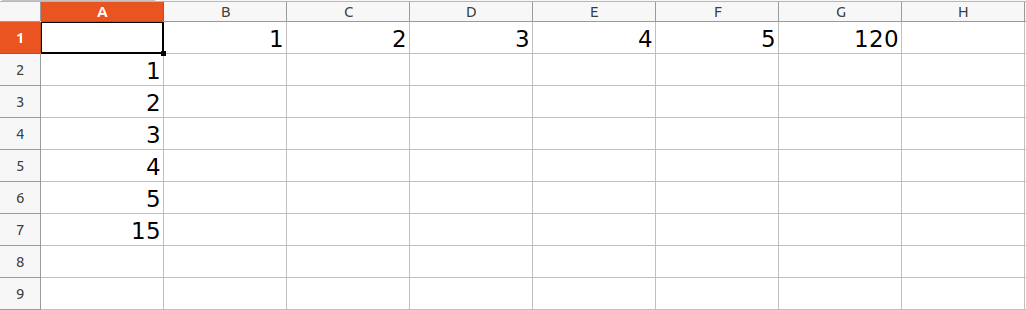XlsxWriter provides a class Chart that acts as a base class for implementing charts. The chart object is created using the add_chart() method. This method also specifies the type of the chart. After creating the chart, the chart is added to the specified cell using the insert_chart() method or it can be set using the set_chart() method.

Syntax:

insert_chart(row, col, chart[, options])

set_chart(chart)

Example 1:

## Python3

 `# import xlsxwriter module ``import` `xlsxwriter `` ` `workbook ``=` `xlsxwriter.Workbook(``'sample.xlsx'``) ``worksheet ``=` `workbook.add_worksheet() `` ` `content ``=` `[``1``, ``2``, ``3``, ``5``, ``3``, ``2``, ``2``] `` ` `# Writing to row and column respectively``worksheet.write_column(``0``, ``0``, content)`` ` `# Creating the chart object of type bar``chart ``=` `workbook.add_chart({``'type'``: ``'column'``})`` ` `# Add a series to the chart``chart.add_series({``'values'``: ``'=Sheet1!\$A\$1:\$A\$7'``})`` ` `# Insert the chart into the worksheet``worksheet.insert_chart(``'C1'``, chart)`` ` `workbook.close()`

Output: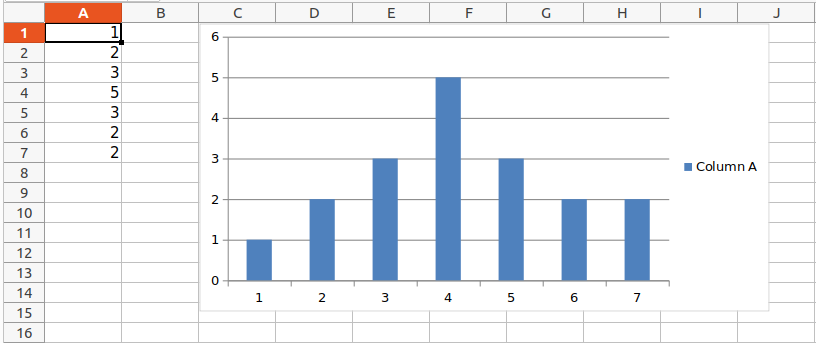Example 2: Adding the line chart with diamond points

## Python3

 `# import xlsxwriter module ``import` `xlsxwriter `` ` `workbook ``=` `xlsxwriter.Workbook(``'sample.xlsx'``) ``worksheet ``=` `workbook.add_worksheet() `` ` `content ``=` `[``1``, ``2``, ``3``, ``5``, ``3``, ``2``, ``2``] `` ` `# Writing to row and column respectively``worksheet.write_column(``0``, ``0``, content)`` ` `# Creating the chart object of type bar``chart ``=` `workbook.add_chart({``'type'``: ``'line'``})`` ` `# Add a series to the chart``chart.add_series({``'values'``: ``'=Sheet1!\$A\$1:\$A\$7'``,``                 ``'marker'``: {``'type'``: ``'diamond'``},})`` ` `# Insert the chart into the worksheet``worksheet.insert_chart(``'C1'``, chart)`` ` `workbook.close()`

Output: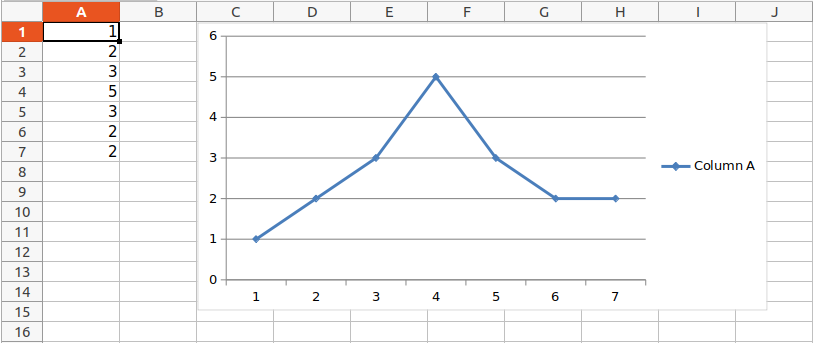Refer to the below articles to get a detailed information about plotting charts using XlsxWriter module.

Tables can be added using the add_table() method. The data parameter of the table is used to specify the data for the cells of the table.. The header_row parameter is used to turn off or on the header row to the table.

Syntax:

Example:

## Python3

 `# import xlsxwriter module ``import` `xlsxwriter `` ` `workbook ``=` `xlsxwriter.Workbook(``'sample.xlsx'``) ``worksheet ``=` `workbook.add_worksheet() `` ` `# Data for the table``data ``=` `[``    ``[``1``, ``2``, ``3``],``    ``[``4``, ``5``, ``6``],``    ``[``7``, ``8``, ``9``],``    ``[``10``, ``11``, ``12``],`` ` `]`` ` `# Creating the Table``worksheet.add_table(``'B2:D5'``, {``'data'``: data})`` ` `workbook.close()`

Output: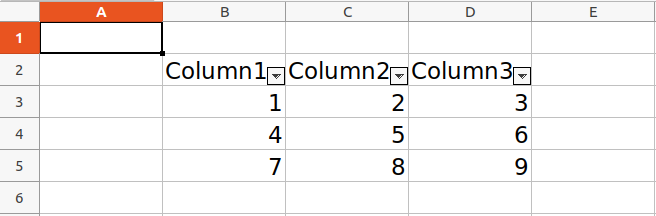## Working with Pandas and XlsxWriter

Pandas write Excel files using the XlsxWriter ot Openpyxl module. This can be used to read, filter, and re-arrange either small or large datasets and output them in a range of formats including Excel. The ExcelWriter() method of the pandas library creates a Excel writer object using XlsxWriter. Then the to_excel() method is used to write the dataframe to the excel.

Example 1:

## Python3

 `# import pandas as pd``import` `pandas as pd`` ` `# Create a Pandas dataframe from some data.``df ``=` `pd.DataFrame({``'Data'``: [``'Geeks'``, ``'For'``, ``'geeks'``, ``'is'``,``                            ``'portal'``, ``'for'``, ``'geeks'``]})`` ` `# Create a Pandas Excel writer``# object using XlsxWriter as the engine.``writer ``=` `pd.ExcelWriter(``'sample.xlsx'``,``                        ``engine``=``'xlsxwriter'``)`` ` `# Write a dataframe to the worksheet.``df.to_excel(writer, sheet_name``=``'Sheet1'``)`` ` `# Close the Pandas Excel writer``# object and output the Excel file.``writer.save()`

Output: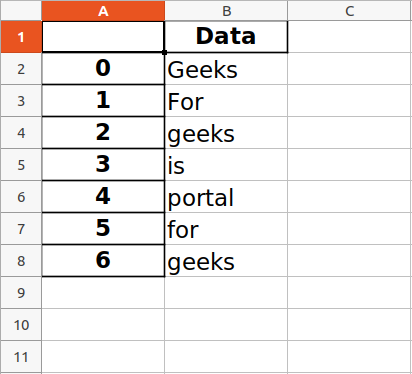Example 2: Writing to multiple dataframes.

## Python3

 `# import pandas as pd``import` `pandas as pd`` ` ` ` `# Create some Pandas dataframes from some data.``df1 ``=` `pd.DataFrame({``'Data'``: [``11``, ``12``, ``13``, ``14``]})``df2 ``=` `pd.DataFrame({``'Data'``: [``21``, ``22``, ``23``, ``24``]})``df3 ``=` `pd.DataFrame({``'Data'``: [``31``, ``32``, ``33``, ``34``]})``df4 ``=` `pd.DataFrame({``'Data'``: [``41``, ``42``, ``43``, ``44``]})`` ` `# Create a Pandas Excel writer object``# using XlsxWriter as the engine.``writer ``=` `pd.ExcelWriter(``'sample.xlsx'``,``                        ``engine``=``'xlsxwriter'``)`` ` `# write and Positioning the dataframes in the worksheet.``# Default position, cell A1.``df1.to_excel(writer, sheet_name``=``'Sheet1'``)``df2.to_excel(writer, sheet_name``=``'Sheet1'``, startcol``=``3``)``df3.to_excel(writer, sheet_name``=``'Sheet1'``, startrow``=``6``)`` ` `# It is also possible to write the``# dataframe without the header and index.``df4.to_excel(writer, sheet_name``=``'Sheet1'``,``             ``startrow``=``7``, startcol``=``4``,``             ``header``=``False``, index``=``False``)`` ` `# Close the Pandas Excel writer object``# and output the Excel file.``writer.save()`

Output: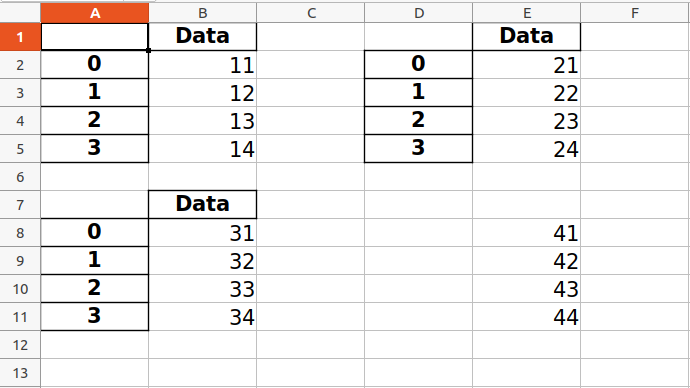Example 3: Converting a Pandas dataframe with datetimes to an Excel file with a default datetime and date format using Pandas and XlsxWriter.

## Python3

 `# import pandas library as pd``import` `pandas as pd`` ` `# from datetime module import``# datetime and date method``from` `datetime ``import` `datetime, date`` ` `# Create a Pandas dataframe from some datetime data.``# datetime(year,month,date,hour,minute,second)``# date(year,month,date)``dataframe ``=` `pd.DataFrame({``    ``'Date and time'``: [datetime(``2018``, ``1``, ``11``, ``11``, ``30``, ``55``),``                      ``datetime(``2018``, ``2``, ``12``, ``1``, ``20``, ``33``),``                      ``datetime(``2018``, ``3``, ``13``, ``11``, ``10``),``                      ``datetime(``2018``, ``4``, ``14``, ``16``, ``45``, ``35``),``                      ``datetime(``2018``, ``5``, ``15``, ``12``, ``10``, ``15``)],`` ` `    ``'Dates only'``: [date(``2018``, ``6``, ``21``),``                   ``date(``2018``, ``7``, ``22``),``                   ``date(``2018``, ``8``, ``23``),``                   ``date(``2018``, ``9``, ``24``),``                   ``date(``2018``, ``10``, ``25``)], })`` ` `# Create a Pandas Excel writer``# object using XlsxWriter as the engine.``# Also set the default datetime and date formats.`` ` `# mmmm dd yyyy => month date year``# month - full name, date - 2 digit, year - 4 digit`` ` `# mmm d yyyy hh:mm:ss => month date year hour: minute: second``# month - first 3 letters , date - 1 or 2 digit , year - 4 digit.``writer_object ``=` `pd.ExcelWriter(``"sample.xlsx"``,``                               ``engine``=``'xlsxwriter'``,``                               ``datetime_format``=``'mmm d yyyy hh:mm:ss'``,``                               ``date_format``=``'mmmm dd yyyy'``)`` ` `# Write a dataframe to the worksheet.``dataframe.to_excel(writer_object, sheet_name``=``'Sheet1'``)`` ` `# Create xlsxwriter worksheet object``worksheet_object ``=` `writer_object.sheets[``'Sheet1'``]`` ` `# set width of the B and C column``worksheet_object.set_column(``'B:C'``, ``20``)`` ` `# Close the Pandas Excel writer``# object and output the Excel file.``writer_object.save()`

Output: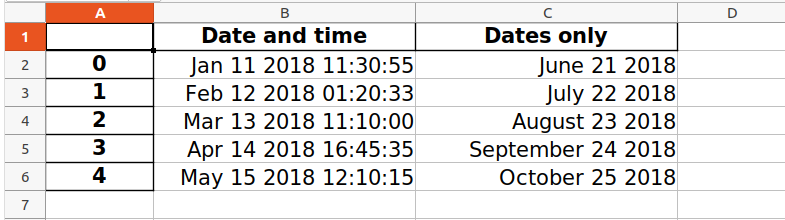Example 4: Converting a Pandas dataframe to an Excel file with a user defined header format using Pandas and XlsxWriter.

## Python3

 `# import pandas lib as pd``import` `pandas as pd`` ` ` ` `data1 ``=` `[``"Math"``, ``"Physics"``, ``"Computer"``,``         ``"Hindi"``, ``"English"``, ``"chemistry"``]`` ` `data2 ``=` `[``95``, ``78``, ``80``, ``80``, ``60``, ``95``]``data3 ``=` `[``90``, ``67``, ``78``, ``70``, ``63``, ``90``]`` ` `# Create a Pandas dataframe from some data.``dataframe ``=` `pd.DataFrame(``    ``{``'Subject'``: data1,``     ``'Mid Term Exam Scores Out of 100'``: data2,``     ``'End Term Exam Scores Out of 100'``: data3})`` ` `# Create a Pandas Excel writer``# object using XlsxWriter as the engine.``writer_object ``=` `pd.ExcelWriter(``"sample.xlsx"``,``                               ``engine``=``'xlsxwriter'``)`` ` `# Write a dataframe to the worksheet.``# we turn off the default header``# and skip one row because we want``# to insert a user defined header there.``dataframe.to_excel(writer_object, sheet_name``=``'Sheet1'``,``                   ``startrow``=``1``, header``=``False``)`` ` `# Create xlsxwriter workbook object .``workbook_object ``=` `writer_object.book`` ` `# Create xlsxwriter worksheet object``worksheet_object ``=` `writer_object.sheets[``'Sheet1'``]`` ` `# Create a new Format object to formats cells``# in worksheets using add_format() method .`` ` `# here we create a format object for header.``header_format_object ``=` `workbook_object.add_format({``    ``'bold'``: ``True``,``    ``'italic'``: ``True``,``    ``'text_wrap'``: ``True``,``    ``'valign'``: ``'top'``,``    ``'font_color'``: ``'green'``,``    ``'border'``: ``2``})`` ` `# Write the column headers with the defined format.``for` `col_number, value ``in` `enumerate``(dataframe.columns.values):``    ``worksheet_object.write(``0``, col_number ``+` `1``, value,``                           ``header_format_object)`` ` `# Close the Pandas Excel writer``# object and output the Excel file.``writer_object.save()`

Output: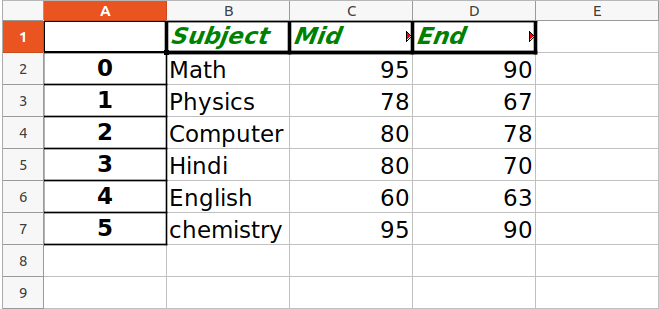### Creating Charts using Pandas and XlsxWriter

Till now we have seen different methods for adding the data to the Excel files using the Pandas and the XlsxWriter module. Now after the data is inserted we can simply create the charts using the add_chart() method as we have seen above.

Example:

## Python3

 `# import pandas library as pd``import` `pandas as pd`` ` `# Create a Pandas dataframe from some data.``dataframe ``=` `pd.DataFrame({``    ``'Subject'``: [``"Math"``, ``"Physics"``, ``"Computer"``,``                ``"Hindi"``, ``"English"``, ``"chemistry"``],``    ``'Mid Exam Score'``: [``90``, ``78``, ``60``, ``80``, ``60``, ``90``],``    ``'End Exam Score'``: [``45``, ``39``, ``30``, ``40``, ``30``, ``60``]})`` ` `# Create a Pandas Excel writer``# object using XlsxWriter as the engine.``writer_object ``=` `pd.ExcelWriter(``'sample.xlsx'``,``                               ``engine``=``'xlsxwriter'``)`` ` `# Write a dataframe to the worksheet.``dataframe.to_excel(writer_object, sheet_name``=``'Sheet1'``)`` ` `# Create xlsxwriter workbook object .``workbook_object ``=` `writer_object.book`` ` `# Create xlsxwriter worksheet object``worksheet_object ``=` `writer_object.sheets[``'Sheet1'``]`` ` `# set width of the B and C column``worksheet_object.set_column(``'B:C'``, ``20``)`` ` `# Create a chart object that can be added``# to a worksheet using add_chart() method.`` ` `# here we create a column chart object .``chart_object ``=` `workbook_object.add_chart({``'type'``: ``'column'``})`` ` `# Add a data series to a chart``# using add_series method.`` ` `# Configure the first series.``# syntax to define ranges is :``# [sheetname, first_row, first_col, last_row, last_col].``chart_object.add_series({``    ``'name'``:     [``'Sheet1'``, ``0``, ``2``],``    ``'categories'``: [``'Sheet1'``, ``1``, ``3``, ``6``, ``3``],``    ``'values'``:     [``'Sheet1'``, ``1``, ``2``, ``6``, ``2``],``})`` ` `# Configure a second series.``chart_object.add_series({``    ``'name'``:     [``'Sheet1'``, ``0``, ``1``],``    ``'categories'``: [``'Sheet1'``, ``1``, ``3``, ``6``, ``3``],``    ``'values'``:     [``'Sheet1'``, ``1``, ``1``, ``6``, ``1``],``})`` ` `# Add a chart title.``chart_object.set_title({``'name'``: ``'Exam Score Distribution'``})`` ` `# Add x-axis label``chart_object.set_x_axis({``'name'``: ``'Subjects'``})`` ` `# Add y-axis label``chart_object.set_y_axis({``'name'``: ``'Marks'``})`` ` `# add chart to the worksheet with given``# offset values at the top-left corner of``# a chart is anchored to cell E2``worksheet_object.insert_chart(``'B10'``, chart_object,``                              ``{``'x_offset'``: ``20``, ``'y_offset'``: ``5``})`` ` `# Close the Pandas Excel writer``# object and output the Excel file.``writer_object.save()`

Output: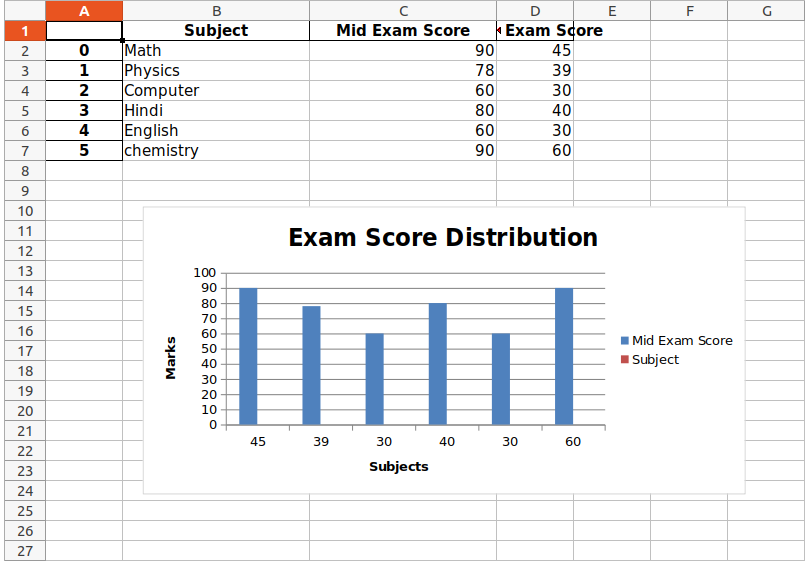Refer to the below articles to get detailed information about working with Xlsxwriter and Pandas.

My Personal Notes arrow_drop_up